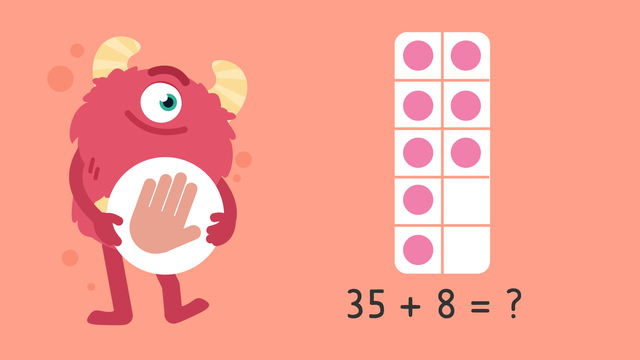# Using Make Ten to Add — Let's PracticeRating

Ø 5.0 / 1 ratings
The authorsTeam Digital

## Basics on the topicUsing Make Ten to Add — Let's Practice

Today we are practicing using make ten to add with Razzi! This video contains examples to help you practice, learn, and become more confident in addition!

## Using Make Ten to Add — Let's Practice exercise

Would you like to apply the knowledge you’ve learned? You can review and practice it with the tasks for the video Using Make Ten to Add — Let's Practice.
• ### How to add using 10 frames.

Hints

When you add 2 or more addends together, you get the "sum"!

When solving word problems, you always want to rewrite the information using numbers and math symbols such as + and =.

This type of written phrase is called an equation.

Solution

Step 1: Write down the equation.

Step 2: Fill in the 10 frame with the smaller addend.

Step 3: Start from the larger addend and then count up using the 10 frame.

• ### Help Mr. Squeaks solve the equation.

Hints

Fill in the 10 frame with the smaller addend.

The addends for this equation are 27 and 8.

If there's not a 10 frame given, you can make your own.

Draw 8 circles or dots on the paper.

Count up from the larger addend using the dots you've just drawn!

Solution

Mr. Squeaks should draw a 10 frame with 8 circles.

Starting from 27, Mr. Squeaks should count the circles in the 10 frame.

So, 27 + 8 = 35

• ### Add using the 10 frames.

Hints

Count up from the larger addend using the 10 frame shown next to the equation.

Let's look at problem #2.

58 + 17 = ?

58 is the larger addend. Count up from 58 using this 10 frame.

Solution

42 + 16 = 58

58 + 17 = 75

39 + 15 = 54

61 + 11 = 72

• ### Solve all the equations.

Hints

For these problems, you should make your own 10 frames.

Start by drawing a rectangle with 10 boxes inside.

Next, color the same number of boxes as the smaller addend in the equation.

If you need to color in more boxes, just draw another 10 frame!

Use this 10 frame to solve problem #1.

Use this 10 frame to solve problem #2.

Use this 10 frame to solve problem #3.

Use this 10 frame to solve problem #4.

Solution

23 + 17 = 40

56 +12 = 68

74 + 18 = 92

67 + 20 = 87

• ### Use the 10 frames to solve the equation.

Hints

Start by identifying the larger number in the equation.

Count up the number of dots in the 10 frame starting from the larger number.

So, the first dot should be counted as 37.

Solution

36 + 13 = 49

Then, use the ten frames to add on 13.

36 + 10 = 46. 46 + 3 = 49.

• ### Solve the word problem.

Hints

The question asks you to add the number of butterflies that Imani saw to the number of butterflies that Mr. Squeaks saw.

Start by writing an addition equation that shows this.

Your equation should look like this:

37 + 19 = ?

Use the 10 frame to help solve the equation.

Solution

Imani and Mr. Squeaks saw 56 butterflies all together.